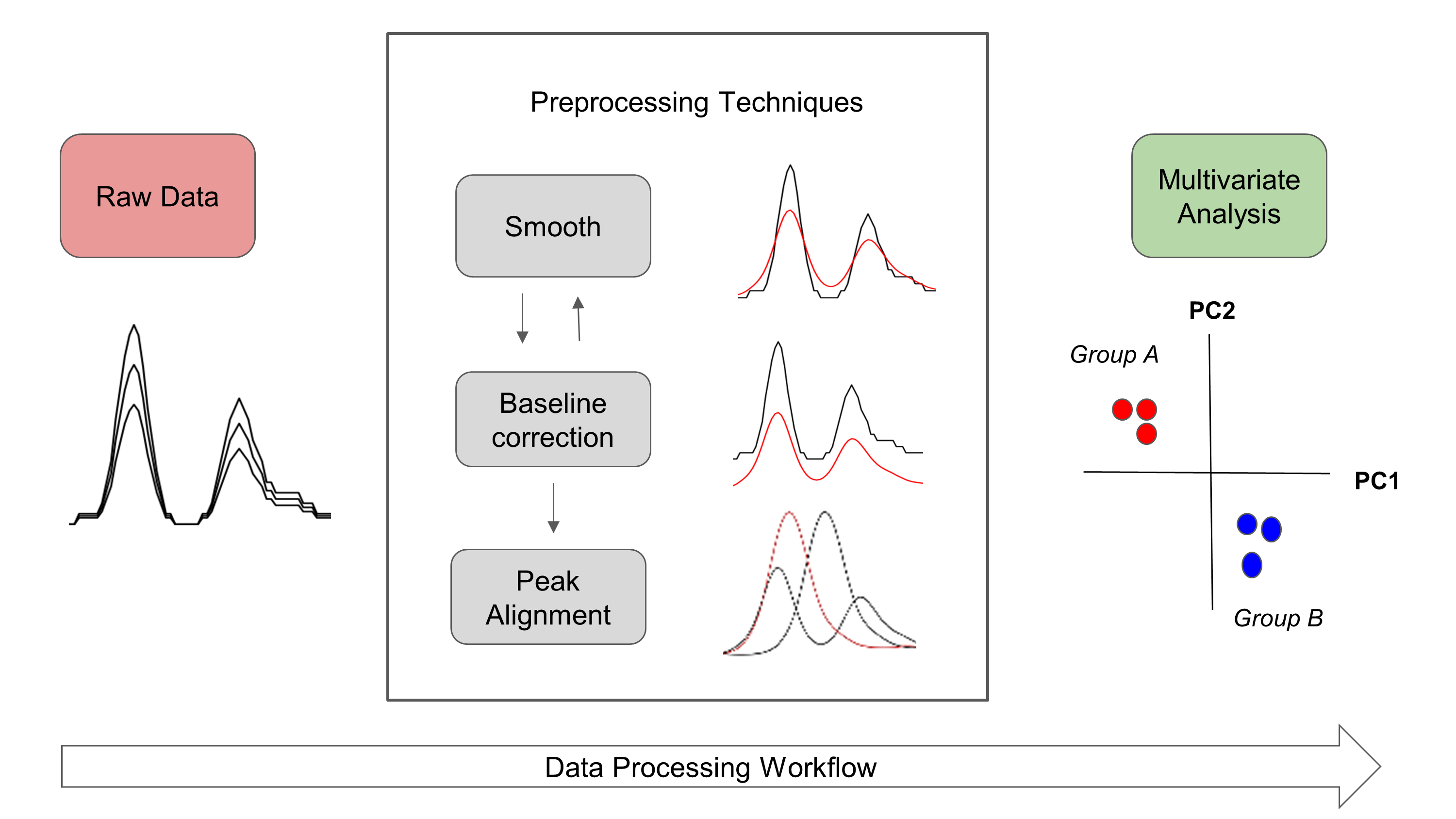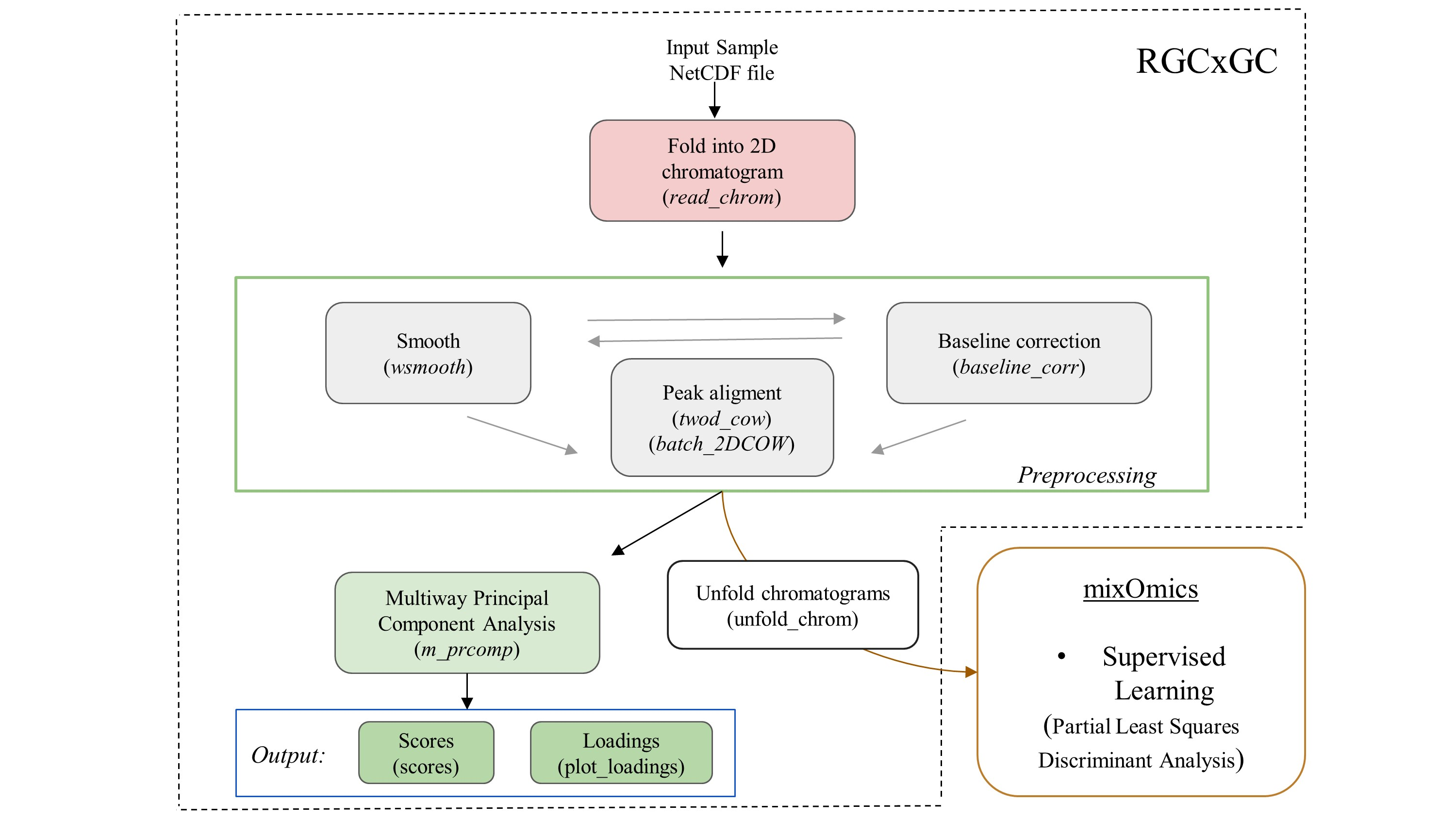# 1 Introduction

This is the vignette to explain the implementation of RGCxGC package. This text presents an end-to-end pipeline for the analysis of comprehensive two dimensional gas chromatography (GCxGC-MS) data. You can access a specific function help through the command help([‘function name’]).

A general workflow about signal preprocessing in chromatography is summarized in Figure 1. Raw chromatographic signals ussually contains undesirable artifacts, such as chemical and instrumental noise. Therefore, noise in the data should be removed prior statistical analysis. Furthermore, noise can be significantly reduced by using preprocessing algorithms, herein smoothing, baseline correction, and peak alignment. Then, in order to reveal differences between groups, multivariate analysis can be performed.Figure 1. Overview of general data processing pipeline in chromatography that is presented in the RGCxGC package.

In the RGCxGC package, first, the raw chromatogram is importing from a NetCDF file and is folded into the two-dimensional Total Intensity Chromatogram (2D-TIC). Next, you can perform three preprocessing methodologies in order to enhace signals, such as smooth, baseline correction, and peak alignment. Basically, smooth enhance the signal to noise ratio (S/N), baseline correction handles column blooding, and peak alignment corrects the retention time shift of the peaks across multiple runs. Finally, you can perform a multiway principal component analysis (MPCA) to look for systematic patterns that distiguish your samples.

## 1.1 Basic workflow

The basic workflow of the RGCxGC package is composed of two main steps, preprocessing and multivariate analysis, after the data is imported (Figure 2).Figure 2. The basic workflow of RGCxGC package. The functions for each step are in parenthesis. The double line between smooth andbaseline correction refers to the interchangeable pathway.

The raw NetCDF file is imported with the read_chrom function, by providing the file name and modulation time that the GCxGC data was acquiered. Next, you can perform preprocessing routines like smoothing and/or baseline correction by using the function wsmooth and baseline_corr, respectively. Then, peak alignment of a single sample against a reference chromatogram can be performed based on the two-dimensional correlation optimized warping (2DCOW) algorithm. Alternatively, mutiple sample alignment can be performed with batch_2DCOW function. After signal preprocessing, MPCA can be performed over the dataset by using the m_prcomp functions. As result of the MPCA, you can acces to the score matrix through scores function, or plot the loading matrix with the plot_loading function. Finally, the MPCA summary can be retrieved with the print function. On the other hand, the RGCxGC package can export the two-dimensional chromatograms in order to perform supervised models. It can be done through unfold_chrom. Supervised models can be done with the mixOmics functions.

# 2 Detailed workflow

## 2.1 Installation

You can install the package in different manners. The most common is to install it from CRAN.

``install.packages("RGCxGC")``

Another way is to install it is through the developer version in github.

``````library(devtools)
install_github("DanielQuiroz97/RGCxGC")``````

Once you have successfully installed the package, you can acces to every functions and data provided by calling the library.

``library(RGCxGC)``
``## Loading required package: RNetCDF``
``## Loading required package: ptw``
``## Loading required package: mixOmics``
``## Loading required package: MASS``
``## Loading required package: lattice``
``## Loading required package: ggplot2``
``````##
## Thank you for using mixOmics!
## Tutorials: http://mixomics.org
## Bookdown vignette: https://mixomicsteam.github.io/Bookdown
## Questions, issues: Follow the prompts at http://mixomics.org/contact-us
## Cite us:  citation('mixOmics')``````
``````##
## Attaching package: 'RGCxGC'``````
``````## The following object is masked from 'package:graphics':
##
##     plot``````
``````## The following object is masked from 'package:base':
##
##     print``````

## 2.2 Importing raw chromatogram

The example data is retrieved form Diagnostic metabolite biomarkers of chronic typhoid carriage stady. You can access to the whole dataset with the identifier MTBLS579 in the MetaboLights database.

You can import the raw chromatogram through the read_chrom function. This function requires at least two parameters: the name of the NetCDF file (name), and the modulation time. This is an adaptation of Skov routine .

``````chrom_08 <- system.file("extdata", "08GB.cdf", package = "RGCxGC")
MTBLS08 <- read_chrom(chrom_08, mod_time = 5)``````
``````## Warning in base_GCxGC(Object = Object, mod_time = mod_time, sam_rate =
## sam_rate, : The last 51 signals will be omitted``````
``````## Warning in matrix(tic, nrow = len_1d, ncol = len_2d): data length  is not
## a sub-multiple or multiple of the number of rows ``````
``````## Retention time ranges:
## 1D (min): 7.98 18.16
## 2D (sec): 0 5
## Acquisition rate: 100``````
``slotNames(MTBLS08)``
``##  "chromatogram" "time"         "name"         "mod_time"``

As we can see, the MTBLS08 object has four slots. The first one correspond to the 2D-TIC chromatogram. The second slot refers to the retention time in the first dimension. The third slot is the name of the NetCDF file, which is first check for validity before importing the chromatogram. Finally, the fourth slot is the modulation time in the second dimension.

## 2.3 Chromatogram visualization

To visualize chromatograms, you can use the plot function. It is built from filled.contour R base function. Since countour plots is a good choice to display non-native GCxGC data . The default function only plots the most intense signals, into a few breaks.

Due to the large variety of metabolite concentrations in a sample, the total ion current can also have large variability in the intensity scale. Therefore, you have to set the number of levels in hundreds. As the number of levels increases, you can obtain a more detailed chromatogram. On the other hand, you can use color palettes presented in the colorRamps package (matlab.like & matlab.like2).

``````# nlevels: Number of levels
# color.palette: The color palette to employ
library(colorRamps)
plot(MTBLS08, nlevels = 100, color.palette = matlab.like2)``````